# Multiples

What is the sum of the multiples of number 7 that are greater than 30 but less than 56?

s =  126

### Step-by-step explanation:

$s=35+42+49=126$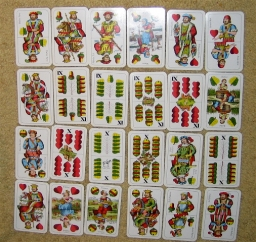Did you find an error or inaccuracy? Feel free to write us. Thank you!## Related math problems and questions:

• I think numberI think number.When I add 841 to it and subtract 157, I get a number that is 22 greater than 996. What number I thinking?
• MultiplesFind all multiples of 10 that are larger than 136 and smaller than 214.
• Czech crowns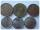Small Petra save money. Grandfather give her 10 crowns. Petra asked his older sister let her calculate how much he has saved up. Sister told her that he has less than 30 crowns. If the sum is divided by three left one crown. If the sum is divided by the f
• On the tripOn the trip were more than 55 children, but less than 65 children. Groups of 7 could be formed, but groups of 8 no. How many children were on a trip?
• Four multiplesFour multiples of 6 he writes the following 12  24  56  72, which is correct?
• Mom and daughter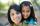Mom is 30 years older than the daughter. What is the age difference between them in 35 years?
• Perpetrator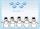The perpetrator is a number that is smaller than the number 80. It is a multiple of five and it is odd. If we added up the tens and ones of this number we get number 8.
• Lesson exercising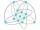The lesson of physical education, pupils are first divided into three groups so that each has the same number. The they redistributed, but into six groups. And again, it was the same number of children in each group. Finally they divided into nine equal g
• Bus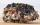On the 4-th stop take on 56 and take off 38 passengers. How many were added (write as positive number) or shrunk (write as negative number) the count of passengers?
• The average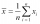The average of one set of 4 numbers is 35. The average of another set of number is 20. The average of the numbers in the two sets is 30. How many numbers are there in the other set?
• How manzBy how many are the product of the numbers 328 and 7 greater than their sum?
• The smallest numberWhat is the smallest number that can be divided by both 5 and 7
• Tomatoes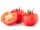There were 2414 crates of tomatoes in the barn, but 735 crates of tomatoes were rotten and had to be thrown out. Joe sold 813 crates and canned 756 crates of tomatoes. How many crates of tomatoes does Joe have left?
• Cakes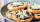On the table was 56 cakes. When Peter went home, leaving them eight times less. How many cakes left?
• Magic numberThe number 135 split to two addends so that one addend was 30 greater than 2/5 the addend.
• Divisible by 5How many three-digit odd numbers divisible by 5, which are in place ten's number 3?
• Storm 3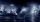If the temperature yesterday was 56 and today is 13 degrees cooler, what is today's temperature?